# NCERT Solutions for Class 8 maths Chapter 1 : rational Numbers Exercise 1.1

In this page we have NCERT Solutions for Class 8 maths Chapter 1 : rational Numbers for Exercise 1.1. Hope you like them and do not forget to like , social share and comment at the end of the page.
Question 1:
Using appropriate properties find: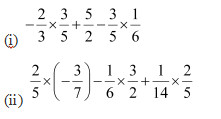1. Using commutativity property of rational number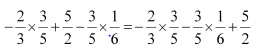Now using Distributivity property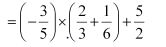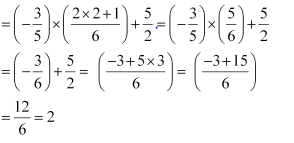(ii) Using commutativity property of rational numbers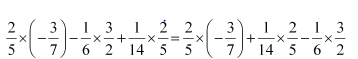Now using Distributivity property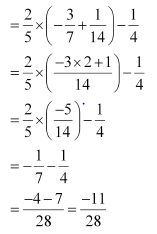Question 2
Write the additive inverse of each of the following:
1. 2/8
2. -5/9
3. -6/-5
4.  2/-9
5. 19/-6

(i) 2/8
(ii) -5/9
(iii) -6/-5 =6/5
(iv)  2/-9=-2/9
(v) 19/-6=  -19/6
Question 3
Verify that - ( - x) = x for.
(i) x =11/15 (ii) x=-13/17
-x=-11/15  As   (11/15)   + (-11/15) =0
The above   also represent that additive inverse of (-11/15) is (11/15) or we can say that
- (-11/15)=11/15
Or x=-(-x)
x=-13/17
-x=-13/17  As   (-13/17)  + (13/17) =0
The above also represent that additive inverse of (13/17) is (-13/17)) or we can say that
- (13/17) = -13/17
Or x=-(-x)
Question 4
Find the multiplicative inverse of the following.
1. -13
2. -13/19
3.  1/5
4. -5/8 X -3/7
5. -1 X -2/5
6.  - 1

1. -13 Multiplicative inverse =-1/13
2. -13/19 Multiplicative inverse =-19/13
3. 1/5 Multiplicative inverse =5
4. -5/8 X -3/7 =15/56 Multiplicative inverse =56/15
5. -1 X -2/5 =2/5 Multiplicative inverse =5/2
-1
Multiplicative inverse =-1
Question 5  :
Name the property under multiplication used in each of the following: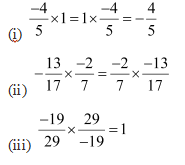1. 1 is the multiplicative identity
2. Commutatively
3. Multiplicative inverse
Question 6:
Multiply 6/13 by the reciprocal of -7/16
Reciprocal of -7/16= -16/7
So (6/13) X (  -16/7) =-96/91
Question 7  Tell what property allows you to compute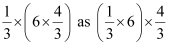Associativity

Question 8
Is   8/9 the multiplicative inverse of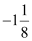Why or why not?=-9/8
Now
(8/9) X   (-9/8)   = -1
So it is not the multiplicative inverse
Question 9   Is 0.3 the multiplicative inverse  of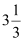Why or why not?=10/3
Now
(3/10) X   (10/3)  =1
So it is the multiplicative inverse
Question 10
Write:
(i) The rational number that does not have a reciprocal.
(ii) The rational numbers that are equal to their reciprocals.
(iii) The rational number that is equal to its negative.

1. 0
2. 1 and -1
3. 0
Question 11 :
Fill in the blanks.
(i) Zero has __________ reciprocal.
(ii) The numbers __________ and __________ are their own reciprocals
(iii) The reciprocal of - 5 is __________.
(iv) Reciprocal of (1/x)   where is x ≠ 0 __________.
(v) The product of two rational numbers is always a __________.
(vi) The reciprocal of a positive rational number is __________.

1. No
2. 1 and -1
3.  -1/5
4. X
5. Rational Number
6. Positive rational number

Reference Books for class 8 Math

Given below are the links of some of the reference books for class 8 Math.

1. Mathematics Foundation Course for JEE/Olympiad : Class 8 This book can take students maths skills further. Only buy if child is interested in Olympiad/JEE foundation courses.
2. Mathematics for Class 8 by R S Aggarwal Detailed Mathematics book to clear basics and concepts. I would say it is a must have book for class 8 student.
3. Pearson Foundation Series (IIT -JEE / NEET) Physics, Chemistry, Maths & Biology for Class 8 (Main Books) | PCMB Combo : These set of books could help your child if he aims to get extra knowledge of science and maths. These would be helpful if child wants to prepare for competitive exams like JEE/NEET. Only buy if you can provide help to the child while studying.
4. Reasoning Olympiad Workbook - Class 8 :- Reasoning helps sharpen the mind of child. I would recommend students practicing reasoning even though they are not appearing for Olympiad.

You can use above books for extra knowledge and practicing different questions.

Note to our visitors :-

Thanks for visiting our website.